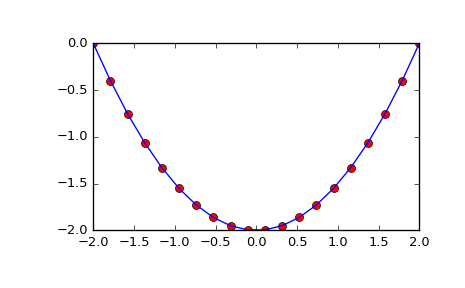# scipy.integrate.cumtrapz¶

scipy.integrate.cumtrapz(y, x=None, dx=1.0, axis=-1, initial=None)[source]

Cumulatively integrate y(x) using the composite trapezoidal rule.

Parameters: y : array_like Values to integrate. x : array_like, optional The coordinate to integrate along. If None (default), use spacing dx between consecutive elements in y. dx : float, optional Spacing between elements of y. Only used if x is None. axis : int, optional Specifies the axis to cumulate. Default is -1 (last axis). initial : scalar, optional If given, uses this value as the first value in the returned result. Typically this value should be 0. Default is None, which means no value at x is returned and res has one element less than y along the axis of integration. res : ndarray The result of cumulative integration of y along axis. If initial is None, the shape is such that the axis of integration has one less value than y. If initial is given, the shape is equal to that of y.

romberg
double integrals
triple integrals
romb
integrators for sampled data
ode
ODE integrators
odeint
ODE integrators

Examples

>>> from scipy import integrate
>>> import matplotlib.pyplot as plt

>>> x = np.linspace(-2, 2, num=20)
>>> y = x
>>> y_int = integrate.cumtrapz(y, x, initial=0)
>>> plt.plot(x, y_int, 'ro', x, y + 0.5 * x**2, 'b-')
>>> plt.show()#### Previous topic

scipy.integrate.trapz

#### Next topic

scipy.integrate.simps